Reach Us+441474556909
Reynolds Number and Spacetime Curvature | OMICS International
Fluid Mechanics: Open Access
All submissions of the EM system will be redirected to Online Manuscript Submission System. Authors are requested to submit articles directly to Online Manuscript Submission System of respective journal.

# Reynolds Number and Spacetime Curvature

Franck Delplace*

ESI group scientific committee 100 Avenue de Suffren, Paris, France

Corresponding Author:
Delplace F
ESI group scientific committee 100 Avenue de Suffren
75015 Paris, France
Tel: +33(0)615319558
E-mail: [email protected]

Received Date: May 30, 2016; Accepted Date: June 10, 2016; Published Date: June 18, 2016

Citation: Delplace F (2016) Reynolds Number and Spacetime Curvature. Fluid Mech Open Acc 3:125. doi:10.4172/fmoa.1000125

Copyright: © 2016 Delplace F. This is an open-access article distributed under the terms of the Creative Commons Attribution License, which permits unrestricted use, distribution, and reproduction in any medium, provided the original author and source are credited.

Visit for more related articles at Fluid Mechanics: Open Access

#### Abstract

The reference length or scale length used in Reynolds number definition is of considerable importance. A quick review of Reynolds number definitions used in batch and continuous flow systems showed that this reference scale can be theoretical or conventional. Using curvature quantity coming from general relativity theory, we showed that Reynolds number could be seen as the ratio of two curvatures. This result could give interesting information for the design of high performances exchangers. Moreover, the use of curvature allowed establishing a relationship between momentum diffusivity and velocity gradient tensor. Applied to general relativity equation, we showed a strong link between gravity theory and hydrodynamics.

#### Keywords

Reynolds; Navier-Stokes; Einstein; Curvature; Viscosity; Reference length; Shear rate; Momentum diffusivity; Gravity; Fluid dynamics

#### Introduction

In fluid mechanics, the Reynolds number is certainly the most important dimensionless quantity used in research and engineering. It is defined as the ratio of momentum to viscous forces and it quantifies the relative importance of these two phenomena for given flow conditions. When viscous forces are predominant, the flow regime is laminar and stream-lines are parallel to each other. Reynolds number values are low and for constant flow conditions in a duct of arbitrary cross section shape, stream-lines velocity varies from zero at the duct walls to a maximum value at the centre of the duct. Turbulent flow regime occurs at higher Reynolds number values when inertia forces become predominant. In that conditions, stream-lines behaviour is more chaotic giving eddies, vortices and other flow instabilities. Between these well-defined flow regimes, there is an often called transition region delimited by critical Reynolds number values. As shown by many authors (Leuliet , Delplace,  Delplace  critical Reynolds number values can vary a lot depending on ducts geometry. Changes of flow regime have a considerable importance for heat and mass transfer industrial applications (heat exchangers, reactors.).

From these well-established results, it is clear that geometry of flow systems is essential to explain and quantify flow phenomena. In the Reynolds number definition, geometry is described through a "reference length" LR sometimes called "scale length". Sometimes, LR can be determined analytically by solving Navier-Stokes equation, this is the well-known case of fully developed laminar flow in a duct of circular cross section shape where LR is the pipe diameter. But when geometries are more complex, LR can be conventional. This is the typical case of mixing tanks where LR is the turbine diameter or a plate heat exchanger having complex flow geometry where LR is defined as twice the maximum gap between two plates (Leuliet , Delplace ).

Recently, fluid mechanics concepts were introduced in theoretical physics for general relativity (Padmanabhan , Delplace ) and quantum mechanics (Delplace ). From these results, it appears that Einstein general relativity equation is similar to Navier-Stokes equation and, if spacetime is considered as fluid; it could be possible to apply Navier-Stokes equation at both astronomic and atomic scales.

General relativity equation tells that spacetime geometry described by its curvature (Einstein tensor) is proportional to stress energy tensor. In every flow system, one can consider we have a spacetime (x, y, z, t in Cartesian coordinates) deformed by stresses produced by the mechanical energy transferred to the liquid by the pumping or mixing device.

The main objective of the present publication is then to revisit the Reynolds number definitions used for batch and continuous flow systems by use of the general relativity concept of curvature available for continuous spacetime medium.

#### Reference Length in Reynolds Number Definitions

Fully established laminar flow of Newtonian fluids in pipes

Solving Navier-Stokes equation in that well known case gives a parabolic velocity profile. For practical use, it is common to use a correlation between the Fanning friction factor f/2 and the Reynolds number Re. This correlation often called friction law takes the following form: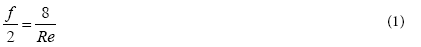with,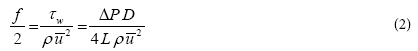and,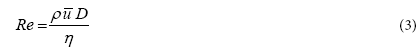In these equations, τw (Pa) is the wall shear stress,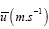is the mean fluid velocity, ρ (kg.m−3 ) is the fluid density, ΔP (Pa) is the pressure drop, D (m) is the pipe diameter L(m), is the pipe length and η (Pa.s) is the dynamic viscosity.

In that perfectly defined case, the reference length found for Reynolds number is the pipe diameter and we have LR=D.

Fully established laminar flow of Newtonian fluids in ducts of arbitrary cross section

In that case, Navier-Stokes equation reduces to the following well known Poisson partial differential equation: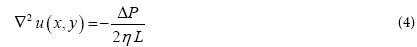u(x, y) is the scalar velocity field in the duct cross section, ΔP (Pa) is the pressure drop L (m), is the pipe length and η (Pa. s) is the dynamic viscosity.

Even for simple geometries like rectangular or triangular ducts, analytical solutions of equation (4) are not simple. Shah et al.  gave a complete review of these solutions for a wide variety of regular ducts. For non regular ducts, only numerical approaches are available and are always an intense field of research (Delplace  Sestak  Sochi ).

As reported by Midoux (1993), for a complex cross section shape, a balance between pressure and friction forces allows determining f/2 (equation 2) in a simple way: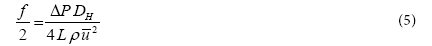DH (m) being the hydraulic diameter defined by: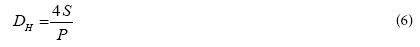S (m2) is the cross section area and P(m) the wetted perimeter.

This definition is of considerable importance because it allows writing friction laws for ducts of arbitrary cross section in the following general form in agreement with equation (1):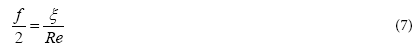As shown analytically and experimentally by many authors (Miller  Leuliet  Delplace ), parameter ξ values depend only on ducts geometries. A good example is the case of rectangular ducts from square duct to well known infinite parallel plates. Solving equation (4), it is possible to calculate for each rectangle of cross section length 2b and cross section width 2a the parameter ξ value as followed: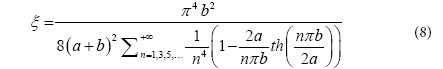In the case of a square duct, numerical calculations gave the well known value 7.1136 (Shah & London ). In the other limit case often called infinite parallel plates where b>>a, the exact solution is: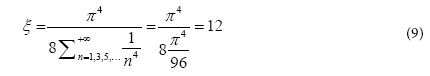Then, for rectangular ducts, for aspect ratio b/a varying from 1 to +∞, ξ values increase from 7,1136 to 12 which is the well-known value for the symmetric case of infinite parallel plates comparable to the other symmetric case of cylindrical duct or pipe giving ξ=8 (equation 1).

The case of cylindrical ducts of arbitrary cross section shape is often called two dimensional flows (2D). The case of three dimensional flows (3D) is much more complex because solving analytically the Navier- Stokes equation is not possible. But the use of dimensionless numbers and particularly the Reynolds number gives powerful tools to engineers.

As a conclusion, for the laminar 2D flow in regular ducts of arbitrary cross section shape, we use LR=DH. This definition of scale length LR can be considered as theoretical on the basis of equation (5).

Fully established laminar flow of Newtonian fluids in complex geometries

As expressed above the case of 3D flows is much more complex than the case of 2D flows. 3D flows are encountered in continuous flow systems in the case of complex shape channels like those built in compact heat exchangers. Because of their extensive use in chemical and food industries, plate heat exchangers are a good example of these complex 3D flow geometries. For laminar flow conditions, plate heat exchangers were studied by many authors and reported in reference encyclopaedias (Cooper  Leuliet  Delplace ).

For plate heat exchangers, all authors used as hydraulic diameter DH=2 e. e being the maximum gap between two plates. ξ values obtained experimentally varied a lot depending on plates geometry or design and on plate heat exchanger flow arrangement. Typical values varied in the range 14 to more than 50.

Another important case of 3D flow is encountered in mixing systems or mixing tanks. In industrial reactors like fermentors, turbines of various designs are used to create 3D flow patterns and obtain a maximum mixing efficiency. A huge amount of scientifical work has been performed to predict power consumption of mixing systems. An accurate review and analyse of this work can be found in Delaplace . Two dimensionless numbers defined below are used to characterise these complex flow systems: the power number Np and the rotational Reynolds number ReR.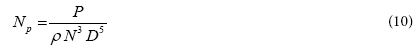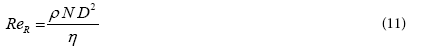In these equations, P (W) is the power consumption of the turbine, N (rad.s−1) its rotational speed, ρ and η being respectively the fluid density and dynamic viscosity.

The complex geometry of mixing systems makes the reference length for both ReR and Np calculation difficult to determine. Consequently, for practical use, D is defined as the turbine diameter even if it is poorly representative of the entire mixing system shape.

The correlation between Np and ReR takes the following form similar to equation (7) obtained for continuous flow systems: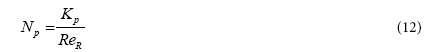Parameter Kp value depends on batch mixing system geometry like parameter ξ value depends on duct geometry.

Couette system used in rheology for viscosity measurements is a simple and good example of equation (12) application. The simple geometry (two cylinders) allows flow equation to be solved analytically and then Kp value to be calculated as a function of cylinders diameters. The narrower is the gap between the two cylinders, the greater is the Kp value. Typical Kp values vary in the range 40 for marine propeller to 300 for helical ribbons and more than 500 for Couette systems .

To conclude, for complex flow systems including batch devices like mixing tanks, we use a conventional reference length for LR in the Reynolds number definition.

Free falling sphere

In the laminar flow regime, the flow equation of a Newtonian liquid around a sphere is given by the following equation (Midoux ):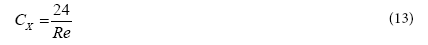with,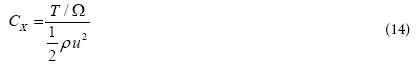and,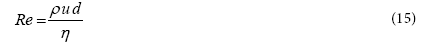In these equations, T (N) is the flow resistance, Ω (m2) is the frontal area, u(m.s-1) is the fluid velocity far from the sphere, and d(m) is the sphere diameter. η and ρ being respectively the dynamic viscosity and the density of the fluid.

Considering that CX can be related to f/2 through the following relationship: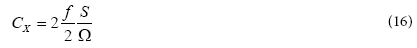Equation (13) takes the same form than equations (1), (7) and (12). This approach is also available for particles having different shapes and the reference length LR used in Reynolds number definition is the diameter in the case of a spherical particle.

Reference length in Reynolds number definition

As expressed in the introduction of this paper, we have shown above, through classical uses of Reynolds number in fluid mechanics, how important is the reference length LR definition. It is clear that when geometry complexity increases, LR changes from a theoretical value (D or DH) to a conventional value (turbine diameter in the case of a mixing tank).

This analysis is based on the hypothesis of laminar flow regime. It is well known that the same Reynolds number definition is used for transition and turbulent flow regimes. Correlations between Reynolds number and Fanning friction factor can be obtained giving the wellknown Blasius law for the turbulent flow in a smooth pipe or others correlations in different geometries (Delplace ).

In turbulent flow regime, it is interesting to consider Kolmogoroff's scales theory. It explains how inertia of large scale eddies is transferred to smaller scales until it reaches a microscopic scale where viscous dissipation occurs and kinetic energy is converted into heat (Midoux ). The Kolmogoroff Reynolds number is defined as followed: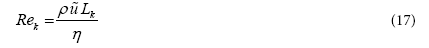Where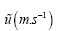is the turbulent fluctuation velocity and LK(m) is the Kolmogoroff scale of turbulence. The value of LK is around 5.10−6m (Midoux ) corresponding approximately to 2.1012 molecules.

To conclude, the reference length used in the Reynolds number definitions is of considerable interest whatever is the flow geometry and even whatever is the flow regime.

#### Others Important Quantities in Friction Laws

As described in §2, friction laws are correlations between the Fanning friction factor and the Reynolds number. The Fanning dimensionless number we called f/2 is the ratio of wall shear stress τw and kinetic energy concentration in the fluid (equation 2). The wall shear stress is related to another important quantity in fluid mechanics: the wall shear rate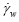through the following relationship: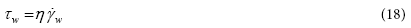Equations (18) and (2) make the wall shear rate for the flow of a Newtonian liquid in a pipe easy to calculate: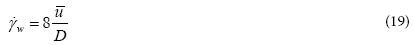being the mean fluid velocity and D(m) the pipe diameter. This theoretical quantity, coming from the parabolic velocity profile obtained for the laminar flow in a pipe was generalized to more complex geometries according to the Reynolds number definition. For a regular pipe of non circular cross section (2D flow), the wall shear rate definition is then: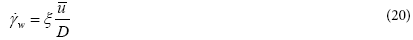For a mixing tank, giving complex 3D flow, another definition of shear rate was necessary. This definition being representative of the entire system can be seen as a mean value: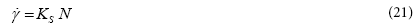In this equation, N (rad.s−1) is the turbine rotational speed and KS a constant representative of the entire mixing system shape. Rotational speed can easily be related to a linear velocity u(m.s-1) through the following well known relationship: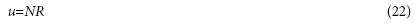This equation is, for a circular motion, the fundamental relationship between angular velocity N, linear velocity u and circle curvature 1/R(m-1). Using equation (22), it is fundamental to notice that equations (19), (20) and (21) take the same form whatever is the flow complexity (2D or 3D). Moreover, it is also very important to consider the coherence between the reference lengths LR used in Reynolds number definitions given above and the shear rate definitions used in this chapter.

#### Spacetime Curvature in Fluids Flow

Fluid flow system as spacetime continuum

Every flow system can be considered as a continuum where small particles of fluid move in a container of fixed geometry. These small particles are analogous to "Atoms of spacetime" introduced by Padmanabhan . Depending on the container geometry and the mechanical energy given to the continuum (the fluid), particles position (x, y, z) in Cartesian coordinates will change with time t.

In the general case, we can consider we have a spacetime continuum delimitated by a rigid container. In this container of given geometry, Navier-Stokes equation describes the motion of the particle M (x, y, z) vs. time t.

The spacetime continuum concept is usual in theoretical physics to describe planets motion through Einstein general relativity theory. But as showed above, the same principles are used in fluid mechanics explaining why Navier-Stokes and Einstein equations can be considered as very similar (Padmanabhan ). In order to use general relativity in fluid mechanics we have to change our definition of dimensions. What we call 2D flow in fluid mechanics is 3D flow for general relativity because time is considered as a length in this theory. For the same reason, 3D flow in fluid mechanics is a 4D flow in general relativity. In the following paragraphs, we will use 3D and 4D descriptions replacing respectively 2D and 3D coordinates previously used.

Spacetime curvature definition

In general relativity theory, one of the most important quantities is the Riemann curvature tensor often called the Einstein tensor Gij (m-2). Its definition given below involved the metric tensor gij (dimensionless), the Ricci tensor Rij (m-2) and the Ricci scalar R (m-2):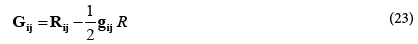This complex equation is the general curvature definition available whatever is the 4D spacetime continuum geometry. Moreover, this quantity giving a local value of spacetime curvature is not well adapted to the case of Reynolds number definition being a global quantity for a given fluid and container system as described in §4.1.

Let us consider the simple case of a circular motion in a 3D spacetime continuum (x, y, t). This is the typical case of what we previously called 2D flow. Scalar curvature C3D (m-1) can simply be defined as the inverse of circle radius R: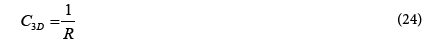As reported in equation (22), C3D is the well known scalar curvature converting a linear velocity into an angular velocity. For the 4D (x, y, z, t) case, we propose to use the well-known scalar curvature of a sphere of radius R: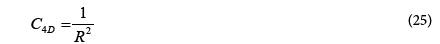The dimension of C4D is m-2 according to the dimension of Gij and we propose to use this simple definition as a mean to characterize the flow geometry in a complex shape container. The use of these equations appears well adapted to circular geometries. As showed in §2.2, the hydraulic diameter is used in Reynolds number definition when duct cross section is not circular. We propose then to use the hydraulic diameter as a mean to characterize curvature of a non-circular duct cross section.

Reynolds number definition using spacetime curvature

For a cylindrical duct of arbitrary cross section, using LR = DH, we obtain the following well known Reynolds number definition: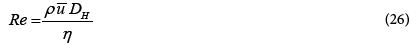Using curvature definitions introduced in §4.2, we have: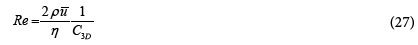This relationship shows that Reynolds number can be considered as the ratio of two curvatures: the curvature of pipe wall C3D and the curvature of the flow itself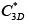due to inertia and viscosity: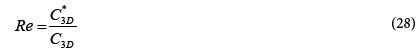Let us use this Reynolds number definition for the case of a pipe of circular cross section in order to illustrate the influence of C3D on Reynolds number value. Developing equation (27) gives the following Reynolds number definition as a function of volumetric flow rate Q(m3.s−1):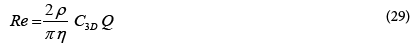For a given flow rate Q of a Newtonian liquid with density ρ and dynamic viscosity η, in a pipe of circular cross section, increasing cross section curvature i.e., decreasing pipe radius will increase Reynolds number value as expected. If you modify pipe wall geometry as described in (Figure 1), you will increase the mean value of wall curvature and then increase the Reynolds number value for the same flow rate and the same liquid physical properties.

It is well known that modified pipes wall geometries improve heat and mass transfers and this new way of considering Reynolds number in terms of curvatures could be of huge interest for the design of high performances geometries. Increasing C3D will enhance heat and mass transfer performances.

Moreover, a comparison between the circular cross section case and others geometries in the same conditions i.e., the same liquid, the same flow rate and the same wall curvature gives an interesting result in term of critical Reynolds number and then in term of laminar flow stability. In equation (29), the numerical value is 2⁄ π≈0.637 for circular cross section. For a square duct, this factor is 1/2 and for an equilateral triangular duct, the same value is 2⁄3 √3 ≈0.385. These values could be representative of experimental critical Reynolds number values reported by Delplace ): 2100 for circular duct, 1150 for square duct and 780 for equilateral triangular duct.

The same approach can be performed in the case of 4D flow encountered in mixing systems. Using equation (11) and equation (25), we obtain: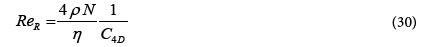This equation is the equivalent of equation (27) for a complex geometry 4D flow system. The use of relationship between linear and angular velocities (equation 22) allows converting equation (27) into equation (30).

To conclude, we showed that Reynolds number can be interpreted as the ratio of two curvatures. Even if the calculation of polygons curvature is always an important field of research in mathematics (Liu  Cantarella  Cufi ), the use of hydraulic diameter in regular ducts of polygonal cross section as a mean to quantify cross section curvature appeared of great interest to compare geometries hydraulic performances using Reynolds number.

Shear rate, momentum diffusivity and spacetime curvature

As reported in §3, shear rate is a very important quantity related to shear stress through equation (20). Another very important parameter is the ratio η ⁄ρ often called kinematic viscosity v in m.s-2. This quantity can be seen as momentum diffusivity (Midoux ).

In equation (28), we defined the flow curvatureas followed:.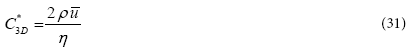Introducing momentum diffusivity in this equation allows the mean velocity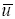to be written as followed: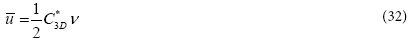The same approach can be done for 4D flow using equation (30) giving: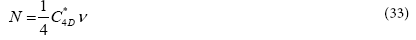Using shear rate definitions reported in §3 give the following definitions for 3D and 4D flows: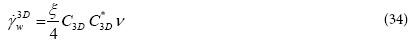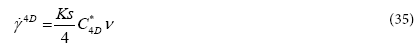Even if these equations describe two different flows: 3D and 4D, they are very similar and of considerable importance. First of all, they show that shear rate or velocity gradient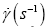is proportional to momentum diffusivity ν(m².s-1) and a volumetric curvature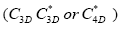in unit m-2.

From a mathematical and theoretical physics point of view, this result allows to write the Einstein tensor Gij in a new way giving the link between Riemann curvature and velocity gradient tensors: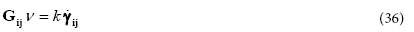To be accurate,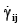is the symmetric part of the velocity gradient tensor often called the rate of strain.

According to equations (34) and (35), constant k value only depends on flow system geometry through ξ and KS values.

The well-known Einstein general relativity equation can then be rewritten as followed: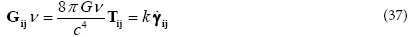and then,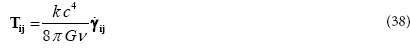.

This relationship between the Einstein stress energy tensor Tij (Pa) and the velocity gradient tensor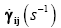is very similar to the rheological equation describing the deformation of a Newtonian fluid without volume modification (Midoux ):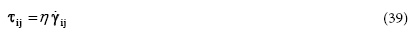τij (Pa) being the stress tensor and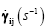the rate of strain tensor. Equation (39) can be considered as the definition of dynamic viscosity (the ratio of stress and velocity gradient tensors) then, the constant term in front of velocity gradient tensor in equation (38) could be interpreted as the definition of a dynamic viscosity in general relativity theory.

It is well known that dynamic viscosity plays a key role in momentum transfer. If η=0, then momentum cannot be transferred between two streamlines in the fluid and then the momentum flux density τij will be null. As a consequence, equation (38) could signify that, if planets motion exists in Einstein curved spacetime, it is due to the existence of a non-zero value of spacetime dynamic viscosity.

At this time where many physicists consider that spacetime could be a fluid, the non-zero dynamic viscosity result given by equation (38) could be of great importance.

Finally, this new way of writing Einstein general relativity equation shows how deep is the relationship between gravity and hydrodynamics.

#### Conclusion

The purpose of this paper was trying to give a new vision of Reynolds number using spacetime curvature. This mathematical quantity is of major interest in theoretical physics because it tells us how space is deformed by energy concentration through the well-known Einstein general relativity theory.

Considering 3D and 4D flow systems, a first result of this approach is a Reynolds number definition as the ratio of two curvatures: the curvature of the flow container and the curvature of the flow itself. This definition could signify a deeper signification of Reynolds number than the well-known ratio of inertia and viscous forces.

Direct practical consequences of this new definition are both the design of modified pipes geometries and the determination of critical Reynolds numbers for transition between laminar and transition flow regimes. The design of modified pipes is of great importance for example in high performances heat exchangers manufacturing. Determination of critical Reynolds number values being also very important for heat transfer applications.

Moreover, because fluid mechanics models are more and more in use in theoretical physics at both astronomic and atomic scales, we tried to find a link between curvature and velocity gradient tensors. This approach gave an important relationship between shear rate, curvature and momentum diffusivity (the ratio of dynamic viscosity and density). Einstein general relativity was then modified in order to obtain an equation similar to the rheological equation describing the deformation of a Newtonian fluid without volume modification. This result allowed the definition of a spacetime dynamic viscosity.

To conclude, recent discoveries like the fluid or liquid nature of Einstein spacetime make essential to build the link between fluid mechanics and cosmology. The famous Reynolds number could play a key role in this approach.

#### References

Select your language of interest to view the total content in your interested language

### Article Usage

• Total views: 10940
• [From(publication date):
July-2016 - Sep 19, 2019]
• Breakdown by view type
• HTML page views : 10656

## Post your commentCan't read the image? click here to refresh
###### Peer Reviewed Journals

Make the best use of Scientific Research and information from our 700 + peer reviewed, Open Access Journals

International Conferences 2019-20

Meet Inspiring Speakers and Experts at our 3000+ Global Annual Meetings

Top